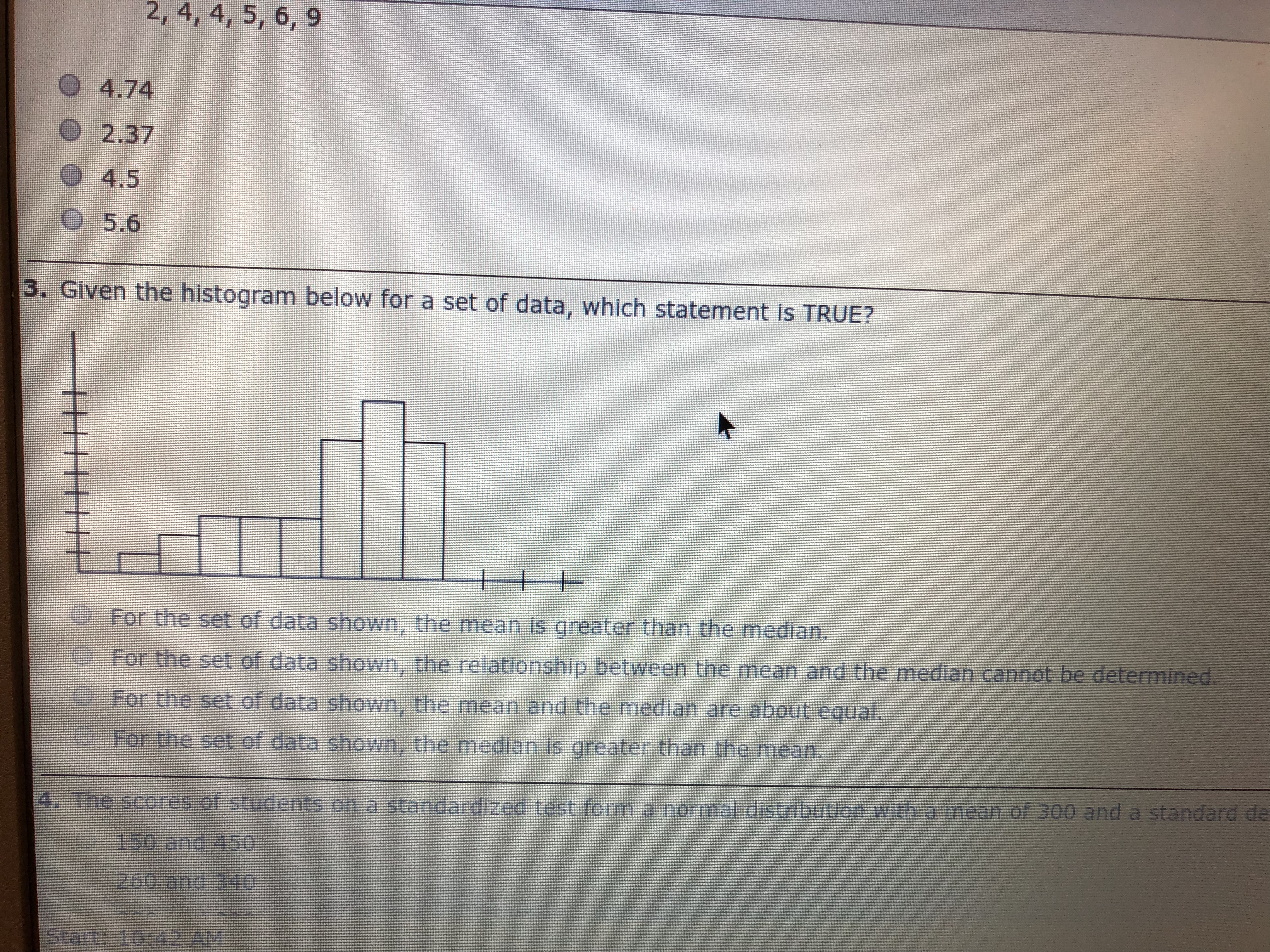# 2, 4, 4, 5, 6, 9 O 4.74 O2.37 O 4.5 O5.6 3. Given the histogram below for a set of data, which statement is TRUE? For the set of data shown, the mean is greater than the median. For the set of data shown, the relationship between the mean and the median cannot be determined. For the set of data shown, the mean and the median are about equal. For the set of data shown, the median is greater than the mean. mean of 300 and a standard de The scores.of students on a standardized test fom.e normal d'stribution with a 150 and 450 260 and 340 Start: 10:42 AM

Question

I guessed that the relationship between the mean and the median cannot be determined.

That was wrong.  I do not Understand what to look for on this diagramhelp_outlineImage Transcriptionclose2, 4, 4, 5, 6, 9 O 4.74 O2.37 O 4.5 O5.6 3. Given the histogram below for a set of data, which statement is TRUE? For the set of data shown, the mean is greater than the median. For the set of data shown, the relationship between the mean and the median cannot be determined. For the set of data shown, the mean and the median are about equal. For the set of data shown, the median is greater than the mean. mean of 300 and a standard de The scores.of students on a standardized test fom.e normal d'stribution with a 150 and 450 260 and 340 Start: 10:42 AM fullscreen

### Want to see this answer and more?

Experts are waiting 24/7 to provide step-by-step solutions in as fast as 30 minutes!*

*Response times may vary by subject and question complexity. Median response time is 34 minutes for paid subscribers and may be longer for promotional offers.
Tagged in
Math
Statistics

### Measures